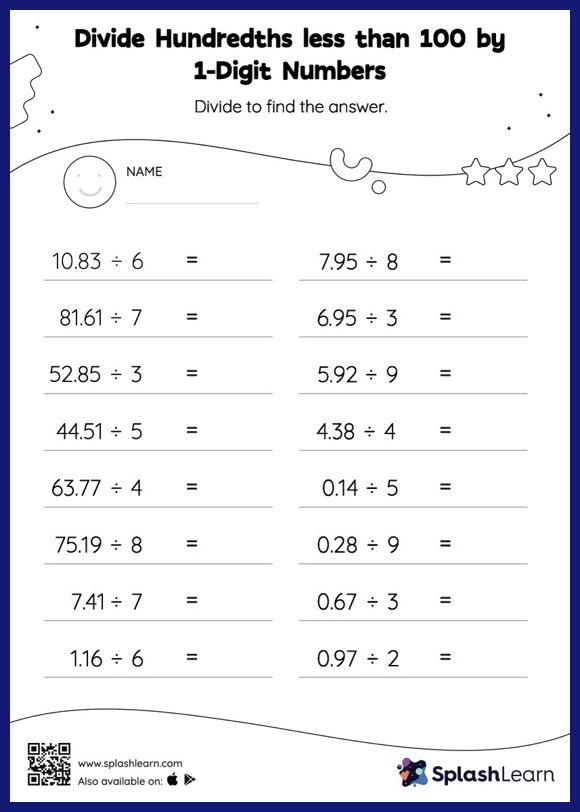# Divide Hundredths less than 100 by 1-Digit Numbers with Remainder: Horizontal Division Worksheet

Home > Divide Hundredths less than 100 by 1-Digit Numbers with Remainder: Horizontal DivisionStudents demonstrate their problem-solving ability as they work on this divide hundredths less than 100 by 1-digit numbers with remainder worksheet. Students divide decimals by 1-digit numbers in the same manner as they would divide whole numbers first. Depending on how many decimal digits are in the dividend, they add a decimal point at the appropriate place in the quotient. Their accuracy with this concept is improved by using the divide hundredths less than 100 by 1-digit numbers with remainder worksheet. In this worksheet, students practice solving problems written in the horizontal format. How numbers are laid out in a problem affects the method a student employs to solve it. Therefore to develop actual fluency and mastery of multiple strategies, students must practice different formats.